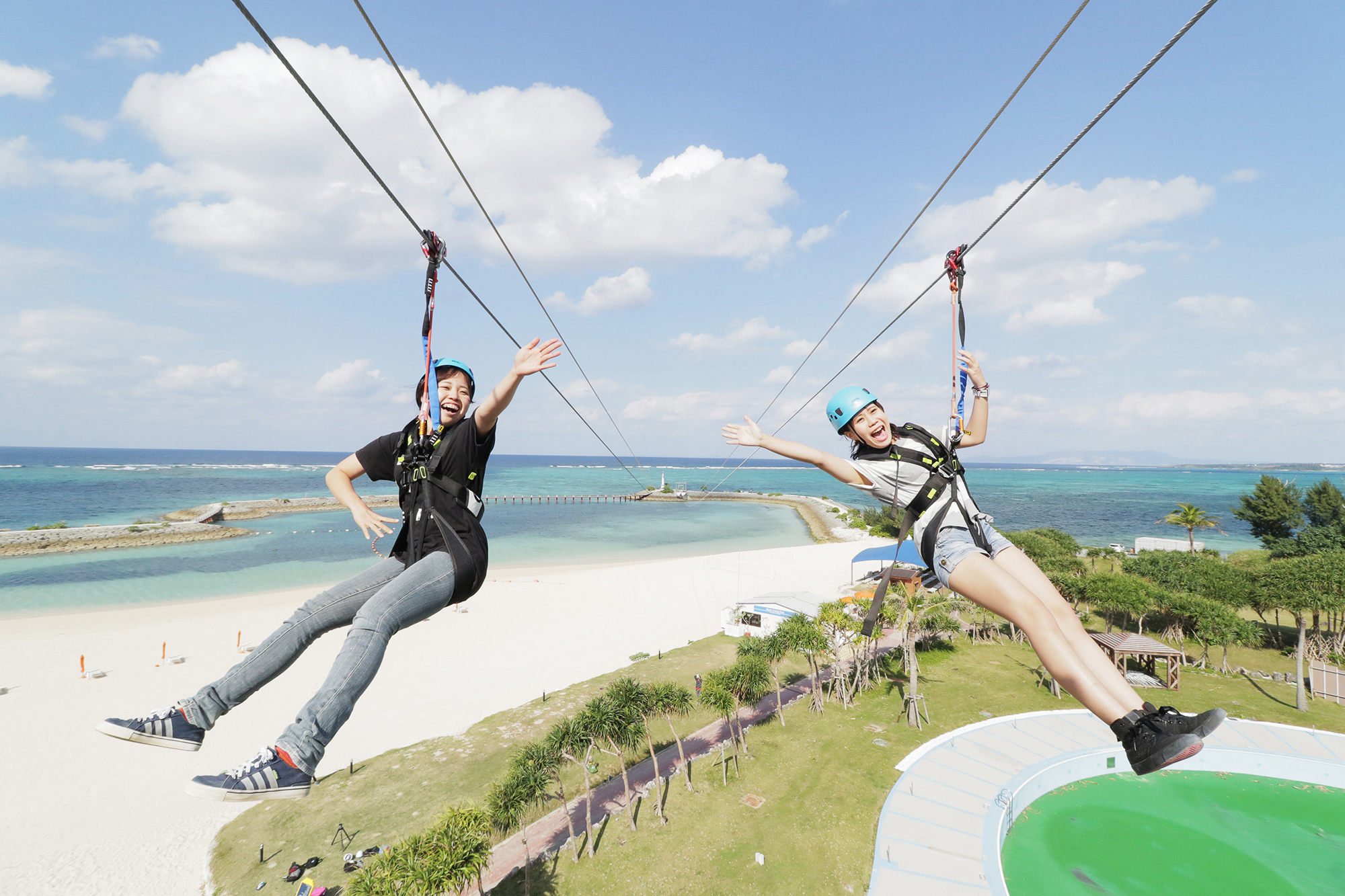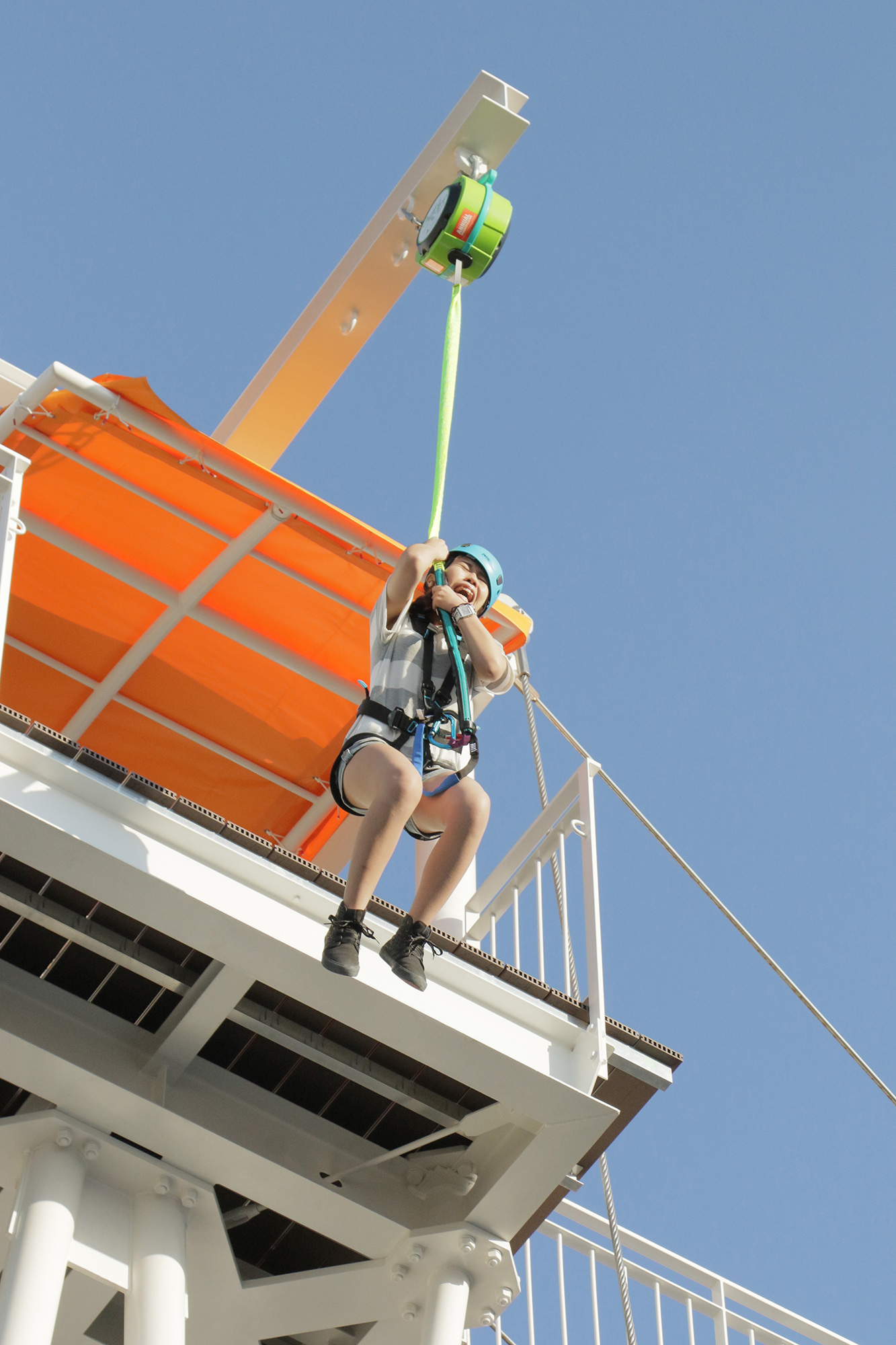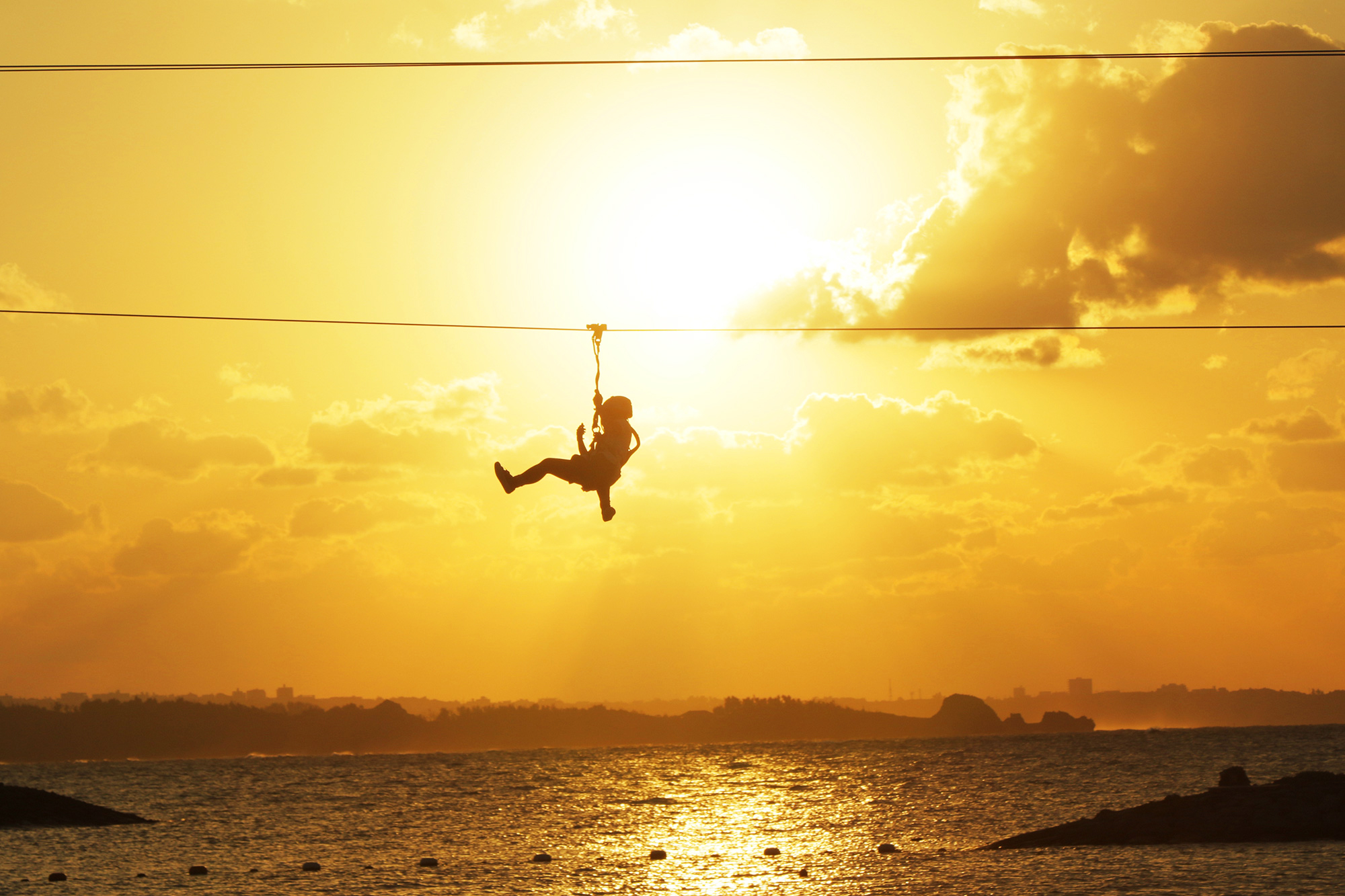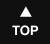### Everything on Okinawa Island from A to Z | Mega Zip & Go Fallメガジップ & ゴーフォール

# Mega Zip & Go FallPanza Okinawa's two exciting activities, Mega Zip & Go Fall are now online at the Sheraton Okinawa Sunmarina Resort 000000000000000000000000000000000000000000000000000000000000000Go Fall is like a (slightly) slower, right-side-up bungee jump. It'll get up up in the morning! 000000000000000000000000000000000000000000000000000000000000000At 250 meters, Mega Zip is the longest zipline in Okinawa and the only one in Japan to run over the ocean

#### 000000000000000000000000000000000000000000000000000000000000000))))))))))))))))))))))))))))))))))))))))))))))))))))))))))))))))))))))))))))))))))))))))))))))))))))))))))))))))))))))))))))))))))))))Take a drive to Onna Village and fill 'er up with sunshine & adrenaline

Introducing Go Fall and Mega Zip, two thrilling new activities from Panza Okinawa at the beautiful Sheraton Okinawa Sunmarina Resort in Onna Village. From four stories up just step off Go Fall's platform for a vertical drop to the ground (just slightly slower than a bungee jump), or take a 30-kilometer-per-hour glide over the hotel grounds, beach and finally the ocean on Mega Zip. At 250 meters, this is the longest zipline in Okinawa and the only one in Japan that runs over the ocean. Prices for Mega Zip are ¥1,200 for hotel guests and ¥1,500 for visitors, while Go Fall costs ¥800 for hotel guests and ¥1,000 for visitors. Both rides can be purchased in a set for ¥1,500 for hotel guests and ¥2,000 for visitors. Some restrictions apply. Participants must be in good health and between 25 and 130 kilograms in weight.